# 4. MOTION IN A PLANE

## 4.1. Position

In Chapter 2 we discussed the motion of an object in one dimension. Its position was unambiguously defined by its distance (positive or negative) from a user defined origin. The motion of this object could be described in terms of scalars. The discussion about motion in two or three dimensions is more complicated. To answer the question "where am I ?" in two dimensions, one needs to specify two coordinates. In three dimensions one needs to specify three coordinates. To specify the position of an object the concept of the position vector needs to be introduced. The position vectoris defined as a vector that starts at the (user defined) origin and ends at the current position of the object (see Figure 4.1). In general, the position vectorwill be time dependent(t). Using the techniques developed in Chapter 3, we can write the position vectorin terms of its components: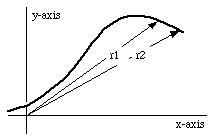Figure 4.1. Definition of Position Vector.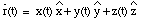Note: In Chapter 2 we got used to plotting the position of the object, its velocity and its acceleration as function of time. In two or three dimensions, this is much more difficult, and most graphs will show for example the trajectory of the object (without providing direct information concerning the time).

## 4.2. Velocity

The velocity of an object in two or three dimensions is defined analogously to its definition in Chapter 2:This equation shows that the velocity of an object in two or three dimensions is also a vector. Again, the velocity vector can be decomposed into its three components: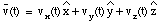The components of(t) can be calculated from the corresponding components of the position vector(t):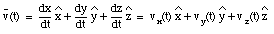We conclude: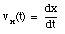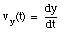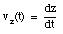## 4.3. Acceleration

The acceleration of an object in three dimensions is defined analogously to its definition in Chapter 2: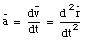This equation shows that the acceleration of an object in two or three dimensions is also a vector, which can be decomposed into three components: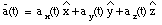The components of(t) can be calculated from the corresponding components of the position vector(t) and the velocity vector(t):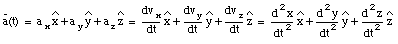We conclude: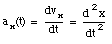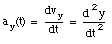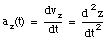Assuming a constant acceleration in the x, y and z direction, we can write down the following equations of motion:

vx(t) = vx0 + ax t

vy(t) = vy0 + ay t

vz(t) = vz0 + az t

The magnitude of the velocity as function of time can be calculated: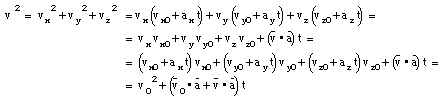If we look at a very small time interval, the change in the velocity vector will be small. In this limit: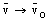The magnitude of the velocity for small time intervals can be written as: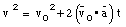From this formula we can conclude: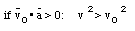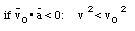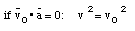Sample Problem 4-2 - 4-4

A rabbit runs across a parking lot on which a set of coordinate axes has, strangely enough, been drawn. The path is such that the components of the rabbit's position with respect to the origin of the coordinate frame are given as function of time by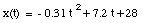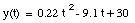The units of the numerical coefficients in these equations are such that, if you substitute t in seconds, x and y are in meters.

The position vector of the rabbit at time t can be expressed as: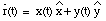From the equations of motion for x(t) and y(t) we can calculate the velocity and acceleration: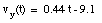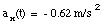The acceleration of the rabbit is constant (independent of time).

The scalar product of the velocity and the acceleration will tell us something about the change of velocity of the rabbit: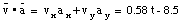From this equation we conclude that for t < 14.6 s (negative scalar product) the rabbit will slow down, while for t > 14.6 s (positive scalar product) the rabbits speed will increase. To check this prediction, we calculate the magnitude of the velocity of the rabbit: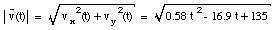The velocity of the rabbit has a minimum at t = 14.6 s.

## 4.4. Projectile Motion

We will start considering the motion of a projectile in 2 dimensions. The coordinate system that will be used to describe the motion of the projectile consist of an x-axis (horizontal direction) and a y-axis (vertical direction). Assuming that we are dealing with constant acceleration, we can obtain the velocity and position of the projectile using the procedure outlined in Chapter 2: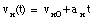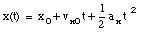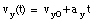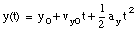where x0 and y0 are the x and y position of the object at t = 0 s, and vx0 and vy0 are the x and y components of the velocity of the object at time t = 0. Note that ax only affects vx and not vy, and ay only affects vy.

In describing the motion of the projectile, we will assume that there is no acceleration in the x-direction, while the acceleration in the y-direction is equal to the free-fall acceleration:

ax = 0

ay = - g = - 9.8 m/s2

In this case, the equations of motion for the projectile are: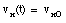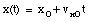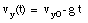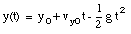The trajectory of the projectile is completely determined by the equations of motion x(t) and y(t). The coordinate system in which we will analyze the trajectory of the projectile is chosen such that x0 = y0 = 0. In this case: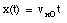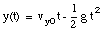The time t can be eliminated from these two equations: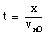Substituting this expression for t into the equation of motion for y, the following relation between x and y can be obtained: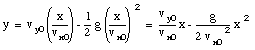We can conclude that the trajectory of the projectile is described by a parabola.

Note: Often, the total velocity v0 of the object at time t = 0 s and the angle [theta] between the direction of the projectile and the positive x-axis is provided. From this information the components of the velocity at time t = 0 s can be calculated: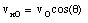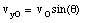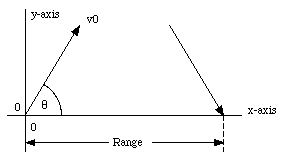Figure 4.2. Projectile Motion.

Example:

Suppose a projectile is launched with an initial velocity v0 and an angle [theta] with respect to the x-axis (see Figure 4.2). What is its range R ?

We start with defining the coordinate system to be used in this problem:

x(t = 0) = x0 = 0

y(t = 0) = y0 = 0

The position of the projectile at any given time t can be obtained from the following expressions: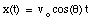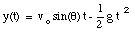At impact, y(t) = 0. The time of impact can therefore be obtained by requiring that y(t) = 0 and solving for t: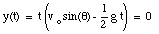This equation has two solutions: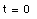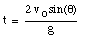The first solution corresponds to the time that the projectile was launched, while the second solution gives us the time that the projectile hits the ground again. The x-coordinate at that time can be obtained by substituting the expression for t into the expression for x(t):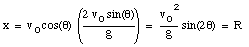The maximum range is obtained when sin(2[theta]) = 1, which corresponds to [theta] = 45deg.. The velocity of the projectile on impact can be calculated using the equations for vx(t) and vy(t):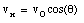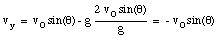Comparing the velocity on impact with the velocity at t = 0, we observe that the velocity component parallel to the x-axis is unchanged, while the component along the y-axis changed sign.

If we look at the equation of the range R, we observe that the for each value of R (less than Rmax) there are two possible launch angles: 45deg. + [Delta][theta] and 45deg. - [Delta][theta] (sin(2[theta]) is symmetric around [theta] = 45deg.). The time of flight for the two cases are however different: a larger launch angle corresponds to a longer time of flight (time of flight is proportional to sin([theta])).

Note: in all our calculations we have neglected air resistance.

Sample Problem 4-7

A movie stunt man is to run across a rooftop and then horizontally off it, to land on the roof of the next building (see Figure 4-16 in Halliday, Resnick and Walker). Before he attempts the jump, he wisely asks you to determine whether it is possible. Can he make the jump if his maximum rooftop speed is 4.5 m/s ?

The coordinate system is chosen such that the origin is defined as the position of the stunt man at the moment he starts his jump from the roof (this is also defined as time t = 0). In this case, the following initial conditions apply:

x0 = 0 m

y0 = 0 m

vx0 = + 4.5 m/s

vy0 = 0 m/s

ax = 0 m/s2

ay = - g

The equations of motion describing the trajectory of the stunt man can now be written as: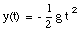The time at which the stunt man has dropped 4.8 m can be calculated from the equation for y(t):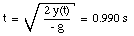The horizontal distance traveled by the stunt man during this time interval can be calculated from the equation of motion for x(t):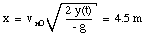However, to reach the next building, the stunt man should have moved 6.2 m horizontally. Unless the stunt man wants to commit suicide, he should not jump.

Example

An antitank gun is located on the edge of a plateau that is 60 m above the surrounding plain. The gun crew sights an enemy tank stationary on the plain at a horizontal distance of 2.2 km from the gun. At the same moment, the tank crew sees the gun and starts to move directly away from it with an acceleration of 0.90 m/s2. If the antitank gun fires a shell with a muzzle speed of 240 m/s at an elevation of 10deg. above the horizontal, how long should the gun crew wait before firing if they are to hit the tank.

Our starting point are the equations of motion of the shell.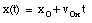(1)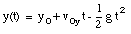(2)

Our coordinate system is defined such that the shell is launched at t = 0 s and its location at that instant is specified by x = 0 m and y = h. Therefore, x0 = 0 m and y0 = h. In order to determine the trajectory of the shell we first determine its time of flight between launch and impact. This time t1 can be obtained from eq.(2) by requiring that on impact y(t1) = 0 m. Thus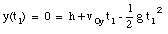(3)

The solutions for t1 are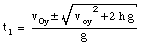(4)

Since the shell will hit the ground after being fired (at t = 0) we only need to consider the positive solution for t1. The range R of the shell can be obtained by substituting t1 into eq.(1):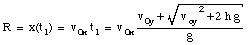(5)

The problem provides the following information concerning the firing of the projectile:

h = 60 m

v0 = 240 m/s

[theta] = 10deg.

Using this information we can calculate v0x and v0y:

v0x = v0 cos([theta]) = 236 m/s

v0y = v0 sin([theta]) = 42 m/s

Substituting these values in eq.(4) and eq.(5) we obtain:

t1 = 9.8 s

R = 2320 m

The distance between the impact point and the original position of the tank is 120 m. The tank starts from rest (v = 0 m/s) and has an acceleration of 0.9 m/s2. The time t2 it takes for the tank to travel to the impact point can be found by solving the following equation: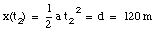(6)

This shows that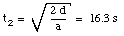(7)

If the shell is fired at the same time that the tank starts to move, the tank will not reach the impact point until (16.3 - 9.8) s = 6.5 s after the shell has landed. This means that the gun crew has to wait 6.5 s before firing the antitank gun if they are to hit the tank.

## 4.5. Circular Motion

Suppose the motion of an object as function of time can be described by the following relations: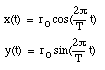(8)

The equations in (8) describe a periodic motion: the position of the object at time t and at time t + T are identical. The period of the periodic motion is obviously T. The path of this object will be circular. This can be easily verified by calculating the distance of this object to the origin: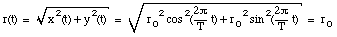(9)

Clearly, the distance of the object to the origin is constant (independent of time). See Figure 4.3. The velocity of the object can be easily calculated using the relations in eq.(8):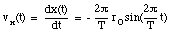(10a)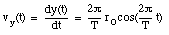(10b)

The direction and magnitude of the velocity vector are: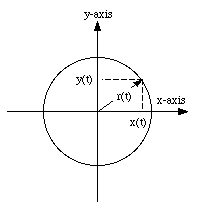Figure 4.3. Definition of trajectory.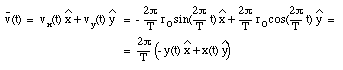(11)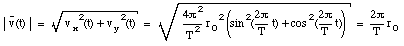(12)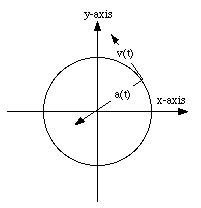Figure 4.4. Direction of velocity and acceleration.

Equation (11) shows that the direction of the velocity is perpendicular to the position vector. In a very similar manner the acceleration of the object can be calculated: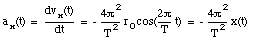(13a)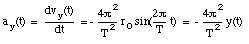(13b)

The direction and magnitude of the acceleration vector are: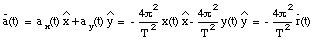(14)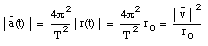(15)

Equation (14) shows that the direction of the acceleration is in the radial direction (opposite to the position vector). See also Figure 4.4.

Note: Equation (12) shows that the magnitude of the velocity of the object is constant (independent of time). Equation (11) shows that only its direction changes with time.

Note: Equation (15) shows that the acceleration is non-zero. It is clear that this non-zero acceleration does not change the magnitude of the velocity, but it changes its direction. The acceleration will be non-zero if either the magnitude or the direction of the velocity changes.

## 4.6. Relative Motion in 1D

The description of the motion of an object depends on the motion of the observer. A man standing along a highway observes cars moving with a velocity of + 55 miles/hour. If our observer would be traveling in one of these cars with a velocity of + 55 miles/hour he would see the other cars moving with a velocity of 0 miles/hour. This demonstrates that the observed velocity of an object depends on the motion of the observer. We will start our discussion with relative motion in one dimension.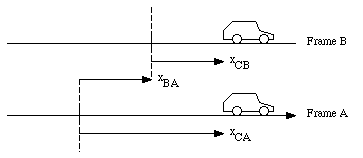Figure 4.5. Relative Motion in One Dimension.

Suppose a moving car is observed by an observer located at the origin of frame A, and by an observer located at the origin of frame B (see Figure 4.5). The snapshot shown in Figure 4.5 shows that at that instant, the distance between observer A and observer B equals xBA. The position of the car measured by observer A, xCA, and the position of the car measured by observer B, xCB, are related as follows:

xCA = xBA + xCB

If we differentiate this equation with respect to time, the following relation is obtained for the velocity of the car measured by observer A and by observer B:

vCA = vBA + vCB

where vCA is the velocity of the car measured by observer A, vCB is the velocity of the car measured by observer B, and vBA is the velocity of observer B measured by observer A. If observer A and observer B do not move with respect to each other (vBA = 0 m/s), the velocity of the car measured by observer A is equal to the velocity of the car measured by observer B.

In a similar fashion, we can obtain a relation between the acceleration of the car measured by observer A and the acceleration measured by observer B:

aCA = aBA + aCB

If the observer in frame B is moving with a constant velocity with respect to the observer in frame A (vBA = constant and aBA = 0 m/s2), the acceleration measured in frame A is equal to the acceleration measured in frame B:

aCA = aCB

Sample Problem 4-12

Alex, parked by the side of an east-west road, is watching car P, which is moving in a westerly direction. Barbara, driving east at a speed vBA = 52 km/h, watches the same car. Take the easterly direction as positive.

a) If Alex measures a speed of 78 km/h for car P, what velocity will Barbara measure ?

This problem involves three cars that are located somewhere along a highway running from east to west. Our coordinate system is chosen such that a positive velocity corresponds to motion towards the east while a negative velocity corresponds to motion towards the west. Alex is sitting in a car parked along the side of the highway (frame A) and Barbara is driving east with a velocity vBA (measured by Alex) equal to + 52 km/h. Both observe a car P. The velocity of P, VPA (measured by Alex), is - 78 km/h (see Figure 4.6). The velocity of car P measured by Barbara can be calculated as follows:

vPA = vBA + vPB

vPA = - 78 km/h

vBA = 52 km/h

vPB = vPA - vBA = (- 78 - 52) km/h = - 130 km/h

The velocity of Alex measured by Barbara can be calculated in a similar manner, and the resulting velocity is - 52 km/h. The corresponding velocity diagram is shown in Figure 4.7.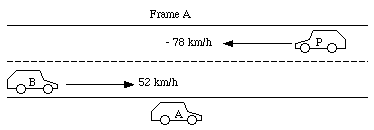Figure 4.6. Velocity Diagram Measured Relative to Alex.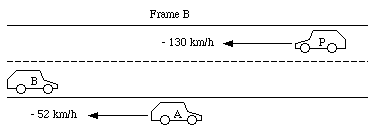Figure 4.7. Velocity Diagram Measured Relative to Barbara.

b) If ALEX sees car P brake to a halt in 10 s, what acceleration (assumed constant) will he measure for it ?

Alex observes that car P brakes to a halt in 10 s. Alex can calculate the acceleration of car P:

v(t) = v0 + a t

The initial conditions are:

v0 = v(t = 0) = - 78 km/h = - 21.7 m/s

The final conditions are:

v(t = 10 s) = 0 m/s

Assuming a constant acceleration, a can be calculated as follows: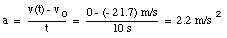c) What acceleration would Barbara measure for the braking car ?

Barbara also observe that car P slows down during this 10 s interval. At time t = 0 she observes car P moving with a velocity equal to v0 = - 130 km/h (- 36.1 m/s). After 10 s, she observes car P moving with a velocity equal to v(t = 10 s) = - 52 km/h (- 14.4 m/s). Barbara can also calculate the acceleration of car P: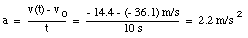Both Alex and Barbara measure the same acceleration of car P. This is what we expected since Barbara moves with a constant acceleration with respect to Alex.

## 4.7. Relative Motion in 2D

The description of the motion of an object in two or three dimensions depends on the choice of the coordinate system. Figure 4.8 shows two reference frames in two dimensions. The vectors rPA and rPB are the position vectors of object P in reference frame A and in reference frame B, respectively. Vector rBA is the position of observer B (located at the origin of reference frame B) in frame A. The position vector of the object P in reference frame B can be obtained from the position vector in reference frame A: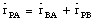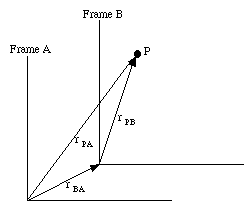Figure 4.8. Reference Frames in Two Dimensions.

The velocity and acceleration of the object P in reference frame B can be obtained by differentiating the position vector of P in reference frame B with respect to time: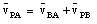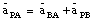If the observer in reference frame B is moving with a constant velocity with respect to an observer in reference frame A, the acceleration of the object P measured by observer A will be the same as the acceleration measured by observer B.

Send comments, questions and/or suggestions via email to wolfs@pas.rochester.edu and/or visit the home page of Frank Wolfs.